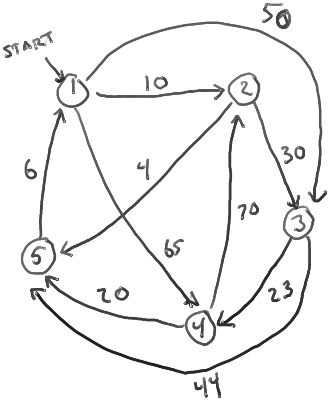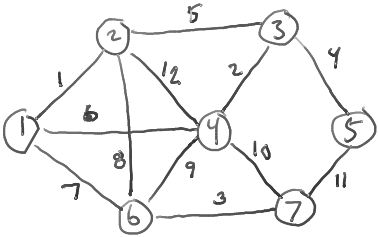# ClassS04CS141/Hwk5

ClassS04CS141 | ClassS04CS141 | recent changes | Preferences

## Hwk5, due Monday, week 9, at start of class

1. Run Dijkstra's algorithm on the following directed graph, starting at vertex 1. After each iteration of the outer loop, show the contents of the queue Q (the vertices in the queue and their keys). What are the final distances (in the distance[] array)?2. The bottleneck of a path is the smallest weight WT(u,v) of any edge (u,v) on the path. Given a directed graph G=(V,E) with edge wts and a source vertex s, define, for each vertex v,
max_bottleneck(s,v) = the maximum, over all paths p from s to v, of the bottleneck of p.
The maximum bottleneck paths problem is (given G, s, and WT), to compute max_bottleneck(s,v) for each vertex v.

(For example, suppose the vertices represent cities, the edges represent roads, and the weight WT(u,v) of an edge (u,v) is the clearance of the lowest bridge on the road from u to v. Then max_bottleneck(s,v) is the height of the largest truck that can get from s to v.)

2A: For each vertex v in the graph for problem 1, what is max_bottleneck(s,v)?

2B: Describe a linear-time algorithm for maximum bottleneck paths that works for acyclic digraphs. Give a high-level description and pseudo-code.

3. A spanning tree of a undirected graph G is a tree (a connected, acyclic subgraph) containing all the vertices of G.

If G has edge weights, the weight of a tree T is the sum of the weights of the edges in the tree T.

The minimum spanning tree problem is to find a spanning tree T (of a graph G with edge weights) of minimum possible weight.3A: What is the weight of the minimum-weight spanning tree in the graph above? What are the edges of the tree?

3B: Claim: in any graph G with edge weights, there is only one spanning tree of minimum total weight. (That is, if T and T' are two different spanning trees, then only one of them can be a minimum spanning tree.)

Prove or disprove this claim.

3C: Claim: in any graph G, the highest-weight edge cannot be in any minimum spanning tree of G.

Prove or disprove this claim.

4. Suppose you have a directed graph G=(V,E) with non-negative vertex weights (that is, every vertex v is assigned a numeric weight WT(v)). You have a start vertex S and a destination vertex T, and you want to find a path from S to T such that the total weight of the vertices along the path is as small as possible.

Describe how you would design an algorithm to do this.

5. Review the algorithm for single-source shortest paths by dynamic programming. (Bellman-Ford algorithm, summarized here.)

Modify this algorithm to instead compute single-source maximum bottleneck paths (defined in problem 2, above.) Describe the high-level idea of your modification, and give pseudo-code too.

ClassS04CS141 | ClassS04CS141 | recent changes | Preferences